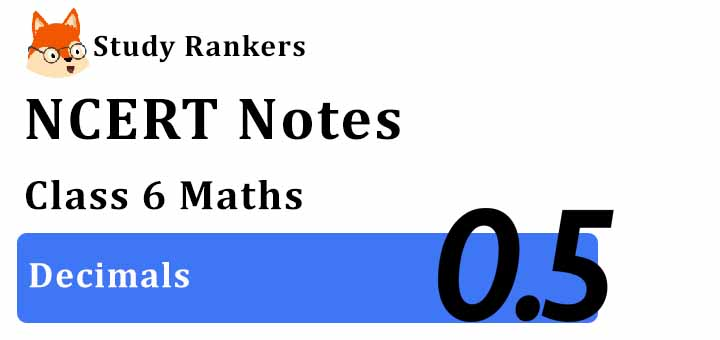# Chapter 8 Decimals Class 6 Notes Maths

Chapter 8 Decimals Class 6 Notes Maths that will be make sure that a student has understood the specifics of every chapter in clear and precise manner. It is quite easy to retain the answers once you are fully aware of the concept thus notes can be beneficial for you. Through these Revision notes for Class 6 students can achieve more marks in the examinations. NCERT Solutions for Class 6 Chapter 8 Maths that can be used to enrich knowledge and make lessons for learners more exciting.Decimals

• Fractions with denominator 10, 100, 1000, etc. (known as decimal fractions) can be written in a form
using a decimal point.

• A decimal has two parts-whole number part and decimal part. These parts are separated by a dot (.). called the decimal point.

Tenths

• Decimal fractions with 10 as denominator.

• One-tenth = 1/10 = 0.1, read as point one.

• Two-tenths = 2/10 = 0.2, read as point two.

• Seven-tenths = 7/10 = 0.7, read as point seven.

Hundredths

• Decimal fractions with 100 as denominator.

• One-hundredths = 1/100 = 0.01, read as point zero one.

• Two-hundredths = 2/100 = 0.02, read as point zero two.

• Seven-hundredths = 7/100 = 0.07, read as point zero seven.

Thousandths

• Decimal fractions with 1000 as denominator.

• One-thousandths = 1/1000 = 0.001 read as point zero zero one.

• Two-thousandths = 2/1000 = 0.002 read as point zero zero two.

• Seven-thousandths = 7/1000 = 0.007. read as point zero zero seven.

Fractions as Decimals

• Fractions can be converted into decimals by writing them in the form with denominators 10.100, 1000 and so on. For example, 5/10 = 0.5, 5/100 = 0.05.

Decimals as Fractions

•  Decimals can be converted into fractions by removing their decimal points and writing 10, 100, etc. in the denominators, depending upon the number of decimal places in the decimals. For example, 0.7 = 7/10, 0.07 = 7/100.

• Convert the given decimals into like decimals.
• Write the addends one under the other in column form, keeping the decimal points of all the addends in the same column and the digits of the same place in the same column.
• Add as in the case of whole numbers.
• In the sum, put the decimal point directly under decimal points in the addends.

Subtraction of Decimals

• Convert the given decimals into like decimals.
• Write the smaller number under the larger one in column form in such a way that the decimal points of
both the numbers are in the same column and the digits of the same place lie in the same column.
• Subtract as we do in case of whole numbers.
• In the difference, put the decimal point directly under the decimal points of the given numbers.

Using Decimals

• Many daily life problems can be solved by converting different units of measurements such as money, length, weight, etc. in the decimal form.

Money

• 100 paise = ₹1
• 1 paise = ₹1/100 = ₹0.01
• 5 paise = ₹5/100 = ₹0.05
• 105 paise = ₹1 + 5 paise = ₹1.05
• 7 rupees 8 paise = ₹7 + ₹0·08 = ₹7.08
• 7 rupees 80 paise = ₹7 + ` 0·80 = ₹7.80

Length

• 10 mm = 1 cm
• 1 mm = 1/10 cm = 0.1 m
• 100 cm = 1 m
• 1 cm = 1/100 m = 0.01 m
• 1000 m = 1 km
• 1 m = 1/1000 = 0.001 km

Weight

• 1000 g = 1 kg
• 1 g = 1/1000 kg = 0.001 kg
• 25 g = 25/1000 kg = 0.025 kg